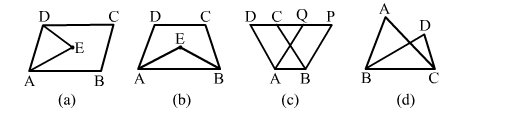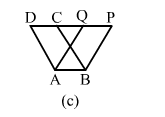# In which of the following figures, you find polynomials on the same base and between the same parallels?

Question:

In which of the following figures, you find polynomials on the same base and between the same parallels?Solution:In this figure, the following polygons lie on the same base and between the same parallel lines:
a) Parallelogram ABCD
b) ​​​Parallelogram ABPQ